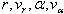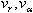# Reference: Array

Model ElementThe Reference_Array model statement specifies a list of variables (which are used by other MotionSolve modeling elements) or constants.

## Description

To specify a list of variables, choose from one of the following types:
• X: This type is used to store the state vector corresponding to the Control_StateEqn and Control_SISO elements.
• Y: This type is used to store the output vector corresponding to the Control_StateEqn and Control_SISO elements.
• U: This type is used to store the input vector corresponding to the Control_StateEqn, Control_SISO and Control_PlantInput elements. The data stored in this type is a list of IDs for Reference_Variable elements that specify the inputs to the modeling elements.
To specify a list of constants, choose the following type:
• IC: This type is used to store a set of numbers primarily to specify initial conditions for Control_StateEqn and Control_SISO elements. You may also use this type to define a set of numbers in the model file and access them from a user-written subroutine.

## Format

<Reference_Array
id                  = "integer"
[ label               = "string" ]
{
type                = "U"
num_element         = "integer"
[ variable_id         = "integer   integer ... integer">
|
usrsub_dll_name     = valid_path_name
usrsub_param_string = "USER([[par_1[,...][,par_n]])"
usrsub_fnc_name     = "custom_fnc_name" >
|
script_name         = valid_path_name
interpreter         = "PYTHON" | "MATLAB"
usrsub_param_string = "USER([[par_1[,...][,par_n]])
usrsub_fnc_name     = "custom_fnc_name" >
]
| type                = "X"
num_element         = "integer" >

| type                = "Y"
num_element         = "integer" >

| type                = "IC"
num_element         = "integer" >
}
!
! The data
!

real        real        real        real        real        real
...         ...         ...         ...         ...         ...
real        real        real        real        real        real
</Reference_Array>

## Attributes

id
Element identification number (integer>0). This number is unique among all the Reference_Array elements.
label
The name of the Reference_Array element.
type
Specifies the type of the Reference_Array being created. Select one from the following:
• X (state variables)
• Y (output variables)
• U (input variables)
• IC (initial conditions)
num_element
The number of input variables being specified when the Reference_Array type is U.
variable_id
List of IDs of the Reference_Variable elements used to define the input array (U).
num_element
The number of elements being specified when the Reference_Array is of type X (state), Y(output), or IC (initial conditions).
usrsub_param_string
The list of parameters that are passed from the data file to the user defined subroutine. Use this keyword only when type = U is selected. This attribute is common to all types of user subroutines/scripts.
usrsub_dll_name
Specifies the path and name of the DLL or shared library containing the user subroutine. MotionSolve uses this information to load the user subroutine ARYSUB in the DLL at run time. Use this keyword only when type = U.
usrsub_fnc_name
Specifies an alternative name for the user subroutine ARYSUB.
script_name
Specifies the path and name of the user written script that contains the routine specified by usrsub_fnc_name.
interpreter
Specifies the interpreted language that the user script is written in. Valid choices are MATLAB or PYTHON.

## Example

A ball placed on a tabletop is allowed to roll with one degree of freedom along the length of the tabletop (no slipping allowed). A motor controls the angle of the beam (alpha). When the angle is changed from the horizontal position, gravity causes the ball to roll along the beam. A full state feedback controller is used to maintain the ball on the beam. See the image below for a schematic of the system.

Linearizing the equations about , the equations governing the ball and controller (shown in red) are:

The parameter c is a function of the mass and inertia properties of the ball, whereas k1, k2, k3, and k4 are properties (gains) of the controller that has been designed. The system has four states:.denote the velocity of the ball and the rate of change of the table angle, respectively. There is one input to the system: a disturbance u to the angular acceleration of the tabletop. There is one output that is being measured: the location of the ball on the tabletop.

Assume c=-7 and k1, k2, k3, and k4 are respectively 1.8E3, 1.0E3, 2.0E3 and 1.0E3. We want to specify the Reference_Arrays associated with this example. Assume that all four states start at zero.

Specifying the initial conditions array:

<Reference_Array
id                  = "102"
type                = "IC"
num_element         = "4">
0.0     0.0     0.0     0.0
</Reference_Array>

Specifying the X array:

<Reference_Array
id                  = "103"
type                = "X"
num_element         = "4">
</Reference_Array>

Specifying the Y array:

<Reference_Array
id                  = "104"
type                = "Y"
num_element         = "1">
</Reference_Array>

Specifying the U array:

<Reference_Array
id                  = "104"
type                = "U"
num_element         = "4"
variable_id         = "1, 2, 3, 4" >
</Reference_Array>

Note that four Reference_Variable elements with IDs 1, 2, 3, and 4 are required to be created somewhere else in the model to define the four inputs. In this example, the first three are set to zero. The fourth represents disturbance due to the tabletop acceleration.